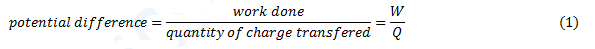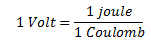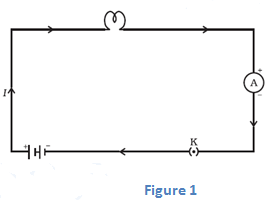# Electric potential, potential difference ,Electric current and electrical circuits|Class 10 electricity Notes

## 4. Electric potential and potential difference

• Charges present in a conductor does not flow from one end to another on their own.
• Electric charges or electrons move in a conductor only if there is a difference of electric pressure, called potential difference, along the conductor.
• This difference of potential may be produced by a battery, consisting of one or more electric cells.
• Potential difference across the terminals of the cell is generated due to chemical reaction within the cell.
• When the cell is connected to a conducting circuit element, the potential difference sets the charges inside the conductor in motion and produces an electric current.
• In order to maintain the current in a given electric circuit, the cell has to expend its chemical energy stored in it.
• The potential difference between two points in an electric field is defined as the amount of work done in moving a unit positive charge from one point to another point. So,• The SI unit of electric potential difference is volt (V)
• The potential difference between two points is said to be one Volt if 1 Joule of work is done in moving 1 Coulomb of electric charge from one point to another. Thus• The potential difference is measured by means of an instrument called the voltmeter.
• The voltmeter is always connected in parallel across the points between which the potential difference is to be measured.
Watch this tutorial for learning about electric potential and potential difference.

### Flow of charge

consider two identical metallic spheres P and N , carrying equal amount of positive and negative charges as shown in this figure.Now we want to take a positive charge from point B to point A. We already know that unlike charges attract each other and  like charges repel each other. So, this positive charge is attracted by the negatively charged sphere N and repelled by the positively charged sphere P.

Here our goal is to move this positive charge from point B to A. So, to move the charge towards point A, you would have to apply the force on this charge towards the left. This is because this positive charge is repelled by the sphere P.

Now you might remember this from class 9 physicsclass 9 physics that work is said to be done when a force produces motion. Here also the force you are applying on positive charge towards left results in the motion of charge towards left.

Since force applied on the charge displaces it in the direction of application of force, the work done is positive. Hence the potential difference VA-VB between the points A and B is positive. And from this we can conclude that VA>VB

Now as you move towards P, the work done increases, so the potential also increases. Now here work done increases because positive charge on P is opposing the motion of charge towards point A. Again if you move towards N the potential decreases. Why does this happens? Think yourself to find answer. So, we can see the potential of P is greater then that of N. In general potential of a positively charged body is taken as higher then that of negatively charged body.

Now a question arises what happens when a free to move charge is placed between these two spheres. In such case a positive charge will move towards the negatively charged sphere and a negative charge will move towards the positively charged sphere. Or we can say that a free positive charge will moves towards lower potential and a free negative charge will move towards higher potential.

Let us now connect these two metal spheres by a metal wire as shown in this figure.After the connection is being made, electrons from negatively charged sphere which is at lower potential will begin to flow towards positively charged sphere which is at higher potential. Again a question arises how long does this flow of charge takes place. Can this go on infinitely. The answer is no. Flow of charges will not takes place infinitely in this arrangement. Eventually , the flow of electrons will balance out the amount of charges on both the spheres.

This means at this point both the spheres have equal amount of charges on them and both of them are at same potential. And this is the point where the flow of electrons stops. So, we can say that potential difference causes the charges to flow. Hence as long as there is a potential difference between two ends of a conductor charges will flow in the conductor.

## Concept Map for electric Potential## 5. Electric current and electrical circuits

• Consider two metallic conducting balls charged at different potential are hanged using a non-conducting insulating wires .Since air is an insulator ,no charge transfer takes place
• Now if we join both the metallic wire using a conducting metallic wire then charge will flow from metallic ball at higher potential to the one at lower potential.
• This flow of charge will stop when the two balls would be at the same potentials.
• If somehow we could maintain the potential between the metallic balls through a cell or battery, we will get constant flow of the charge in metallic wire, connecting the two conducting balls
• This flow of charge in metallic wire due to the potential difference between two conductors used is called electric current.
• So, Electric current is expressed by the amount of charge flowing through a particular area in unit time.
• In other words, it is the rate of flow of electric charges (electrons) in a conductor (for example copper or metallic wire).
• If a net charge Q, flows across any cross-section of a conductor in time t, then the current I, through the cross-section isThe S.I. unit of electric current is Ampere (A)
• When 1 Coulomb of charge flows through a cross-section of conductor in 1 second then current flowing through the conductor is said to be 1 Ampere.
• Current is measured by an instrument called ammeter. It is always connected in series in a circuit through which the current is to be measured.
• A continuous and closed path of an electric current is called an electric circuit. For example figure given below shows a typical electric circuit comprising a cell, an electric bulb, an ammeter A and a plug key K.Note that the electric current flows in the circuit from the positive terminal of the cell to the negative terminal of the cell through the bulb and ammeter
• The conventional direction of electric current is from positive terminal of the cell to the negative terminal through the outer circuit.
• Or we can say that conventional direction of electric current is in the direction of the flow of positive charged carriers.

## Electric Current Concept MapGiven below are the links of some of the reference books for class 10 Science.

You can use above books for extra knowledge and practicing different questions.

### Practice Question

Question 1 Which among the following is not a base?
A) NaOH
B) $NH_4OH$
C) $C_2H_5OH$
D) KOH
Question 2 What is the minimum resistance which can be made using five resistors each of 1/2 Ohm?
A) 1/10 Ohm
B) 1/25 ohm
C) 10 ohm
D) 2 ohm
Question 3 Which of the following statement is incorrect? ?
A) For every hormone there is a gene
B) For production of every enzyme there is a gene
C) For every molecule of fat there is a gene
D) For every protein there is a gene

Note to our visitors :-

Thanks for visiting our website.